## 3.3.2 Contour-Ordered GREEN's Function

To express the field operators in the interaction representation an operator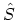is defined (see Appendix B.4) and applied for calculating the GREEN's functions as in Section 3.1.1. The time in (3.8) is taken over the interval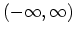. The state at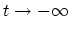is well defined as the ground-state of the non-interacting system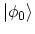. The interactions are turned on slowly. At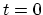the fully interacting ground state is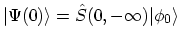. The state at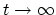must be defined carefully. If the interactions remain on, then this state is not well described by the non-interacting ground state. Alternatively, one could require that the interactions are turned off at large times, which returns the system to the ground-state.

SCHWINGER  suggested another method of handling the asymptotic limit. He proposed that the time integral in theoperator has two parts; one goes from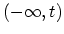while the second goes from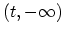. The integration path is a contour, which starts and ends at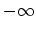. The advantage of this method is that one starts and ends the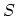operator expansion with a known state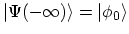. Instead of the time-ordering operator (B.21), a contour-ordering operator can be employed. The contour-ordering operator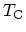orders the time labels according to their order on the contour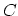. Under equilibrium condition the contour-ordered method gives results that are identical to the time-ordered method described in Section 3.1.1. The main advantage of the contour-ordered method is in describing non-equilibrium phenomena using GREEN's functions. Non-equilibrium theory is entirely based upon this formalism, or equivalent methods.

Any operator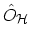in the HEISENBERG picture can be transformed into the interaction picture (see (B.13))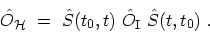(3.19)

Analogous to the derivation of (B.24), it can be shown that theoperator is given by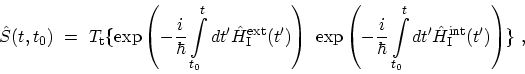(3.20)

where the operators are in the interaction representation. The ordinary time-ordering can also be written as ordering along contour branches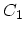and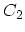as depicted in Fig. 3.1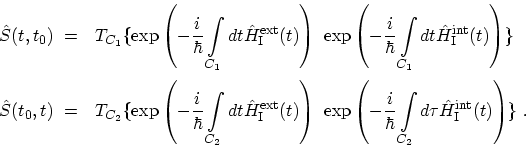(3.21)

By combining two contour branches,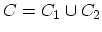, (3.19) can be rewritten as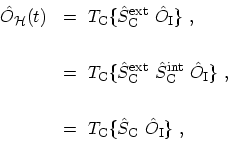(3.22)

where,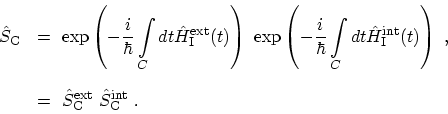(3.23)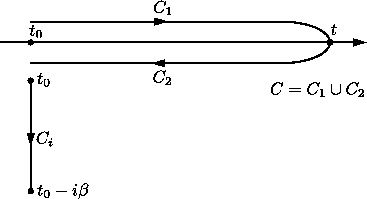In equation (3.17)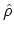describes the equilibrium state of the system before the external perturbation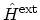is turned on. Interactions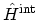, which are switched on adiabatically at, are present in. However, to apply WICK's theorem (Section 3.4.1), one has to work with non-interacting operators. A methodology similar to the MATSUBARA theory can be applied to express the many-particle density operatorin terms of the single-particle density operator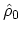, see Appendix B.5. If the contour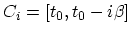is chosen (Fig. 3.1), then (B.34) takes the form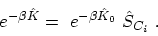(3.24)

Therefore, (3.17) can be rewritten as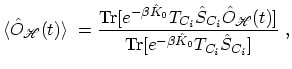(3.25)

Using the relations (3.22) and (3.25), the GREEN's function in (3.18) becomes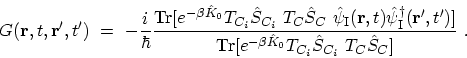(3.26)

The twofold expansion of the density operator and the field operators may conveniently be combined to a single expansion. The two contours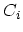andcan be combined together,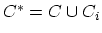(Fig. 3.2), and a contour-ordering operator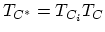, which orders along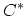, can be introduced. Hence, a point onis always earlier than a point on. Furthermore, we define an interaction representation with respect to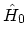onand with respect to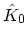on. Therefore, the GREEN's function in (3.18) is given by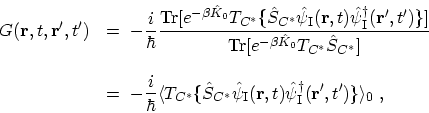(3.27)

where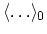represents the statistical average with respect to. From here we assume that all statistical averages are with respect toand drop the 0 from the brackets.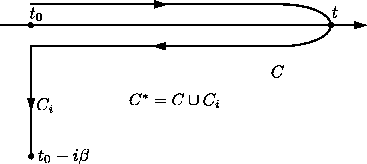M. Pourfath: Numerical Study of Quantum Transport in Carbon Nanotube-Based Transistors